# Math Olympiad Test: Statistics- 1 - Class 9

Test Description

## 10 Questions MCQ Test Mathematics Olympiad for Class 9 - Math Olympiad Test: Statistics- 1

Math Olympiad Test: Statistics- 1 for Class 9 2023 is part of Mathematics Olympiad for Class 9 preparation. The Math Olympiad Test: Statistics- 1 questions and answers have been prepared according to the Class 9 exam syllabus.The Math Olympiad Test: Statistics- 1 MCQs are made for Class 9 2023 Exam. Find important definitions, questions, notes, meanings, examples, exercises, MCQs and online tests for Math Olympiad Test: Statistics- 1 below.
 1 Crore+ students have signed up on EduRev. Have you?
Math Olympiad Test: Statistics- 1 - Question 1

### The range of the following ungrouped data will be: 30, 32, 45, 54, 74, 78, 108, 112, 66, 76, 86, 41, 14, 15, 35, 112.

Detailed Solution for Math Olympiad Test: Statistics- 1 - Question 1

Range = uppermost value – lowest value
= 112 – 14
= 98

Math Olympiad Test: Statistics- 1 - Question 2

### The marks of 40 students in final exam obtained by students of class 9 is given below: 8, 18, 12, 6, 8, 16, 12, 5, 23, 2, 16, 23, 2, 10, 20, 12, 9, 7, 6, 5, 3, 5, 13, 21, 13, 15, 20, 24, 1, 7, 21, 16, 13, 18, 23, 7, 3, 18, 17, 16. Q. The number of students in the class interval 5 – 10 are:

Detailed Solution for Math Olympiad Test: Statistics- 1 - Question 2

5 – 10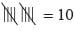Math Olympiad Test: Statistics- 1 - Question 3

### The class marks distribution are: 25, 26, 27, 31, 36, 41, 46, 51, 57, 59 Q. The lower limit of first class interval will be:

Detailed Solution for Math Olympiad Test: Statistics- 1 - Question 3

Lower limit =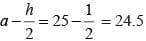Math Olympiad Test: Statistics- 1 - Question 4

Let ‘l’ be the lower limit of a class interval in a frequency distribution and ‘m’ be the mid-point of the class. Then the upper limit of the class is:

Detailed Solution for Math Olympiad Test: Statistics- 1 - Question 4

Given that, the lower class limit of a class interval is l and the mid-point of the class is m. Let u be the upper class limit of the class interval. Therefore, we have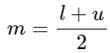⇒ l +u = 2m
⇒ u = 2m - 1
Thus the upper class limit of the class is (2m - l).

Math Olympiad Test: Statistics- 1 - Question 5

The mid value of a class interval is 14 and the class size is 2. The lower limit of the class is:

Detailed Solution for Math Olympiad Test: Statistics- 1 - Question 5

Lower limit = mid-value –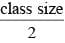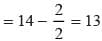Math Olympiad Test: Statistics- 1 - Question 6

A frequency polygon is constructed by plotting frequency of the class interval and the

Detailed Solution for Math Olympiad Test: Statistics- 1 - Question 6

A frequency polygon is constructed by plotting frequency of the class interval and mid value of the class.

Math Olympiad Test: Statistics- 1 - Question 7

Ogives are the graphical representation of

Detailed Solution for Math Olympiad Test: Statistics- 1 - Question 7

Cumulative histograms, also known as ogives, are graphs that can be used to determine how many data values lie above or below a particular value in a data set. An ogive (oh-jive), sometimes called a cumulative frequency polygon, is a type of frequency polygon that shows cumulative frequencies.

Math Olympiad Test: Statistics- 1 - Question 8

Total number of students (prob - 14) are:

Detailed Solution for Math Olympiad Test: Statistics- 1 - Question 8

Total number of students
= 4 + 10 + 16 + 20 + 16
= 66

Math Olympiad Test: Statistics- 1 - Question 9

If the mean of x1, x2, x3 ……., xn isand 5 is added to each number, the new mean will be:

Detailed Solution for Math Olympiad Test: Statistics- 1 - Question 9

Given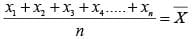⇒ if 5 is added to every number, then,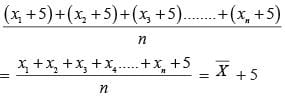Math Olympiad Test: Statistics- 1 - Question 10

The mean of 10 numbers is 16. If two consecutive numbers are excluded, the new mean is 18. The sum of the excluded numbers is:

Detailed Solution for Math Olympiad Test: Statistics- 1 - Question 10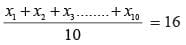⇒ x1 + x2 …… + x10 = 16 ……. (i)
Let x2 and x3 are removed, then,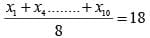⇒ x1 + x4 + ….. + x10 = 144 …….(ii)

Now, Eq. (i) – (ii)

x2 + x3 = 160 – 144 = 16

## Mathematics Olympiad for Class 9

23 videos|82 docs|43 tests